# S2E3 - What are Isotopes? Atomic Structure and Isotope Examples

What the heck are "isotopes?" What's the definition of an isotope? How are they different?

But first, we must introduce the atom and its components by quickly discussing Modern Atomic Structure...

## A Brief Introduction to Modern Atomic Structure

This topic is covered in great detail in SECTION 7 - Quantum Mechanical View of the Atom and Periodicity.

Today we only need to quickly mention electrons, protons, and neutrons before moving on to our main topic, Isotopes.

### Atoms have 3 components:

Electron (e-     9.11 x 10-31 kg     rel. charge =  -1
Proton (p+       1.67 x 10-27 kg     rel. charge =  +1
Neutron (n0)     1.67 x 10-27 kg     rel. charge =   0

The electron's mass is 10,000 times smaller than the mass of a proton or neutron. Protons and neutrons are the two subatomic particles that reside in the nucleus.

In fact, they are the nucleus.

The nucleus (protons and neutrons) accounts for "all" of an atom's mass.  Electrons' contributions to mass are insignificant.

=====

*Question ➞  If all atoms are composed of electrons, protons, and neutrons, why do different atoms have different properties?...

The answer lies in the number and arrangement of electrons, which interact with other electrons from other atoms when combining to form molecules.

=====

## Isotopes

Isotopes = atoms of an element that have the same number of protons, but different numbers of neutrons.

Isotopes can be represented in 2 notations:

1.  nuclear symbol notation
2.  hyphen notation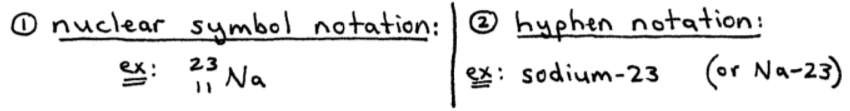Both isotope notations tell you the number of electrons, protons, and neutrons.  Let's take a closer look at nuclear symbol notation...

### Nuclear Symbol Notation

In nuclear symbol notation, the symbol of the element (X) is written.

Then, write a "superscript number to the left" - this is the mass number (A).

We also write a "subscript number to the left" - this is the atomic number (Z).

➞ Mass Number (A) = the sum of protons and neutrons.
➞ Atomic Number (Z) = the total number of protons.

Here's a visual of that: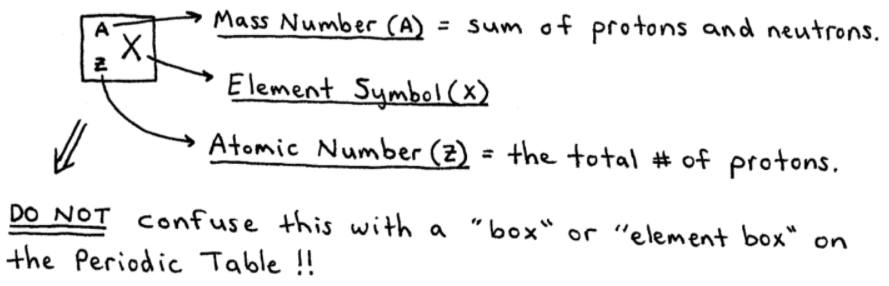-----

### Isotopes Examples

Practice makes perfect, right?  So let's do a few isotopes example problems where we have to write specific isotope notations and/or find the numbers of electrons, protons, and neutrons.

ex:  Write "sodium-23" (hyphen notation) in nuclear symbol notation.
_____
answer:  sodium is Na, and Na has atomic number = 11.  This is Z, and the 23 is our mass number, A.  So we have,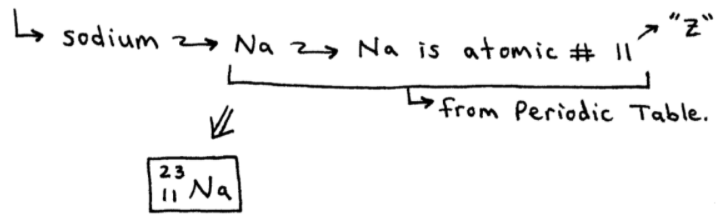-----

ex:  How many electrons, protons, and neutrons does the isotope sodium-23 have?
_____
answer:  you see the "23" ?  That will never be an answer!!  Because it's the sum of both protons and neutrons!!

That said, we have: sodium, which is Na, which means 11 protons.  We can assume it's a neutral atom, so we also have 11 electrons.

23 - 11 = 12 neutrons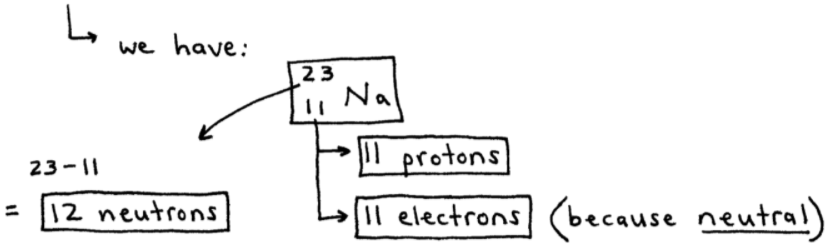-----

ex:  How many electrons, protons, and neutrons are in each of the following isotopes?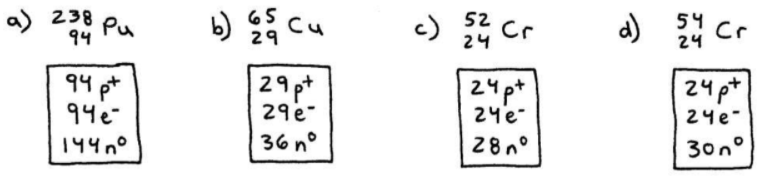-----

ex:  How many electrons, protons, and neutrons are in the following two isotopes?

a.   79Br                      b.   239Pu

Where's "Z" ?

Sometimes Z is left out because it's "understood."  It would be redundant to include it.

For example in (a)  ➞  Bromine is Br  ➞  atomic number = 35  ➞  Z = 35.
_____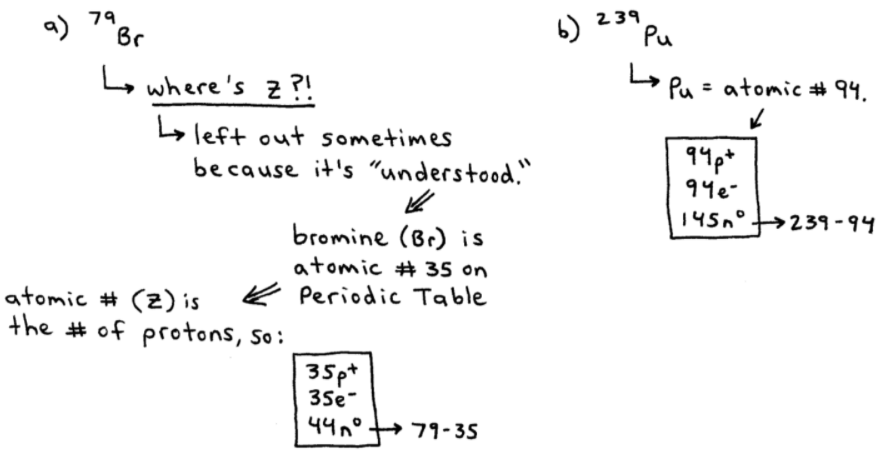=====

As we get closer to learning how to name covalent and ionic compounds, it's best to now move away from Isotopes and begin talking a little bit about Molecules and Ions.

## Molecules and Ions

The forces that hold two or more atoms together are called chemical bonds.  There are two types of chemical bonds:

1. Covalent Bonds
2. Ionic Bonds

Let's just introduce them both here today...

### Covalent Bonds

covalent bonds = when 2 atoms share electrons to form a molecule.  Check out methane as an example:-----

### Ionic Bonds

ions = atoms that have positive or negative charges.

ionic bonds = bonds formed between 2 ions.

ex:  NaCl is not a molecule!  It's an ionic compound.  Here's what the NaCl ionic bond looks like...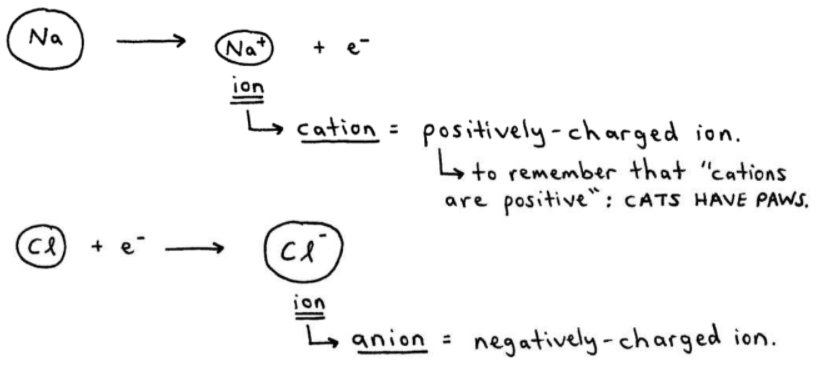Notice that the cation is smaller than it's parent atom, and the anion is larger than its parent atom.

cation = postively-charged ion ("cats have paws")

anion = negatively-charged ion.

Now, we can see below how the cation (Na+) and the anion (Cl-) are attracted to each other through a +/- electrostatic interaction: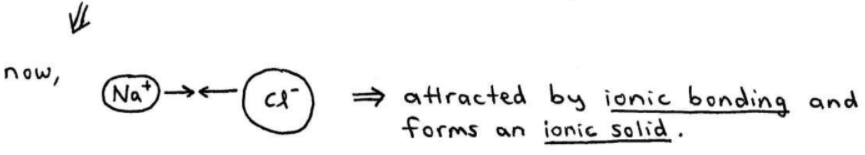=====

If you've enjoyed the first 3 video blog entries on SECTION 2 - Atoms, Molecules, and Ions...

The next one covers the Periodic Table, Metals vs. Nonmetals, and How to Name Ionic Compounds...